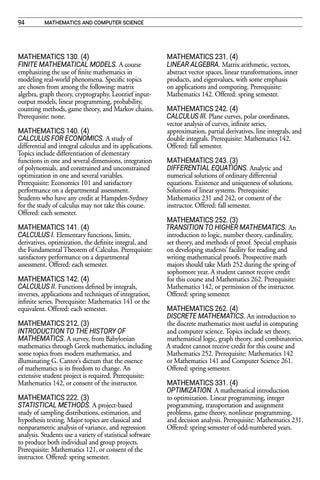94

mathematics and computer science

MATHEMATICS 130. (4) FINITE MATHEMATICAL MODELS. A course emphasizing the use of finite mathematics in modeling real-world phenomena. Specific topics are chosen from among the following: matrix algebra, graph theory, cryptography, Leontief inputoutput models, linear programming, probability, counting methods, game theory, and Markov chains. Prerequisite: none. MATHEMATICS 140. (4) CALCULUS FOR ECONOMICS. A study of differential and integral calculus and its applications. Topics include differentiation of elementary functions in one and several dimensions, integration of polynomials, and constrained and unconstrained optimization in one and several variables. Prerequisite: Economics 101 and satisfactory performance on a departmental assessment. Students who have any credit at Hampden-Sydney for the study of calculus may not take this course. Offered: each semester. MATHEMATICS 141. (4) CALCULUS I. Elementary functions, limits, derivatives, optimization, the definite integral, and the Fundamental Theorem of Calculus. Prerequisite: satisfactory performance on a departmental assessment. Offered: each semester. MATHEMATICS 142. (4) CALCULUS II. Functions defined by integrals, inverses, applications and techniques of integration, infinite series. Prerequisite: Mathematics 141 or the equivalent. Offered: each semester. MATHEMATICS 212. (3) INTRODUCTION TO THE HISTORY OF MATHEMATICS. A survey, from Babylonian mathematics through Greek mathematics, including some topics from modern mathematics, and illuminating G. Cantorâ&#x20AC;&#x2122;s dictum that the essence of mathematics is its freedom to change. An extensive student project is required. Prerequisite: Mathematics 142, or consent of the instructor. MATHEMATICS 222. (3) STATISTICAL METHODS. A project-based study of sampling distributions, estimation, and hypothesis testing. Major topics are classical and nonparametric analysis of variance, and regression analysis. Students use a variety of statistical software to produce both individual and group projects. Prerequisite: Mathematics 121, or consent of the instructor. Offered: spring semester.

MATHEMATICS 231. (4) LINEAR ALGEBRA. Matrix arithmetic, vectors, abstract vector spaces, linear transformations, inner products, and eigenvalues, with some emphasis on applications and computing. Prerequisite: Mathematics 142. Offered: spring semester. MATHEMATICS 242. (4) CALCULUS III. Plane curves, polar coordinates, vector analysis of curves, infinite series, approximation, partial derivatives, line integrals, and double integrals. Prerequisite: Mathematics 142. Offered: fall semester. MATHEMATICS 243. (3) DIFFERENTIAL EQUATIONS. Analytic and numerical solutions of ordinary differential equations. Existence and uniqueness of solutions. Solutions of linear systems. Prerequisite: Mathematics 231 and 242, or consent of the instructor. Offered: fall semester. MATHEMATICS 252. (3) TRANSITION TO HIGHER MATHEMATICS. An introduction to logic, number theory, cardinality, set theory, and methods of proof. Special emphasis on developing studentsâ&#x20AC;&#x2122; facility for reading and writing mathematical proofs. Prospective math majors should take Math 252 during the spring of sophomore year. A student cannot receive credit for this course and Mathematics 262. Prerequisite: Mathematics 142, or permission of the instructor. Offered: spring semester. MATHEMATICS 262. (4) DISCRETE MATHEMATICS. An introduction to the discrete mathematics most useful in computing and computer science. Topics include set theory, mathematical logic, graph theory, and combinatorics. A student cannot receive credit for this course and Mathematics 252. Prerequisite: Mathematics 142 or Mathematics 141 and Computer Science 261. Offered: spring semester. MATHEMATICS 331. (4) OPTIMIZATION. A mathematical introduction to optimization. Linear programming, integer programming, transportation and assignment problems, game theory, nonlinear programming, and decision analysis. Prerequisite: Mathematics 231. Offered: spring semester of odd-numbered years.